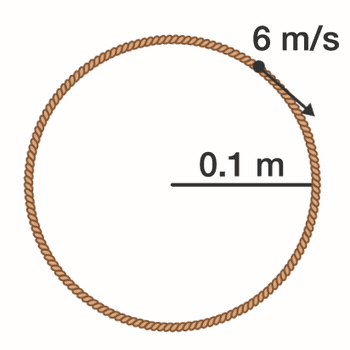# Tension in A Rotating Loop?A rope loop of radius $r = \SI[per-mode=symbol]{0.1}{\meter}$ and mass $m = 50\pi\,\text{g}$ rotates on a frictionless table such that the tangential velocity of any point on the loop is $v_\textrm{tangential}=\SI[per-mode=symbol]{6}{\meter\per\second}.$

Find the tension in the loop (in Newtons).

×